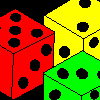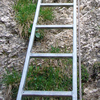# Resources tagged with: Mathematical reasoning & proof

Filter by: Content type:
Age range:
Challenge level:

### There are 89 results

Broad Topics > Mathematical Thinking > Mathematical reasoning & proof### Take Three Numbers

##### Age 7 to 11 Challenge Level:

What happens when you add three numbers together? Will your answer be odd or even? How do you know?### Cows and Sheep

##### Age 7 to 11 Challenge Level:

Use your logical reasoning to work out how many cows and how many sheep there are in each field.### 9 Weights

##### Age 11 to 14 Challenge Level:

You have been given nine weights, one of which is slightly heavier than the rest. Can you work out which weight is heavier in just two weighings of the balance?### Making Pathways

##### Age 7 to 11 Challenge Level:

Can you find different ways of creating paths using these paving slabs?##### Age 5 to 11 Challenge Level:

Who said that adding couldn't be fun?##### Age 7 to 11 Challenge Level:

Three dice are placed in a row. Find a way to turn each one so that the three numbers on top of the dice total the same as the three numbers on the front of the dice. Can you find all the ways to do. . . .### Square Subtraction

##### Age 7 to 11 Challenge Level:

Look at what happens when you take a number, square it and subtract your answer. What kind of number do you get? Can you prove it?### Tower of Hanoi

##### Age 11 to 14 Challenge Level:

The Tower of Hanoi is an ancient mathematical challenge. Working on the building blocks may help you to explain the patterns you notice.### One O Five

##### Age 11 to 14 Challenge Level:

You can work out the number someone else is thinking of as follows. Ask a friend to think of any natural number less than 100. Then ask them to tell you the remainders when this number is divided by. . . .### Online

##### Age 7 to 11 Challenge Level:

A game for 2 players that can be played online. Players take it in turns to select a word from the 9 words given. The aim is to select all the occurrences of the same letter.### Reasoning: the Journey from Novice to Expert (article)

##### Age 5 to 11

This article for primary teachers suggests ways in which we can help learners move from being novice reasoners to expert reasoners.### Always the Same

##### Age 11 to 14 Challenge Level:

Arrange the numbers 1 to 16 into a 4 by 4 array. Choose a number. Cross out the numbers on the same row and column. Repeat this process. Add up you four numbers. Why do they always add up to 34?### More Marbles

##### Age 11 to 14 Challenge Level:

I start with a red, a blue, a green and a yellow marble. I can trade any of my marbles for three others, one of each colour. Can I end up with exactly two marbles of each colour?### Marbles

##### Age 11 to 14 Challenge Level:

I start with a red, a green and a blue marble. I can trade any of my marbles for two others, one of each colour. Can I end up with five more blue marbles than red after a number of such trades?### Elevenses

##### Age 11 to 14 Challenge Level:

How many pairs of numbers can you find that add up to a multiple of 11? Do you notice anything interesting about your results?### Clocked

##### Age 11 to 14 Challenge Level:

Is it possible to rearrange the numbers 1,2......12 around a clock face in such a way that every two numbers in adjacent positions differ by any of 3, 4 or 5 hours?### Sticky Numbers

##### Age 11 to 14 Challenge Level:

Can you arrange the numbers 1 to 17 in a row so that each adjacent pair adds up to a square number?### 1 Step 2 Step

##### Age 11 to 14 Challenge Level:

Liam's house has a staircase with 12 steps. He can go down the steps one at a time or two at time. In how many different ways can Liam go down the 12 steps?### Tri-colour

##### Age 11 to 14 Challenge Level:

Six points are arranged in space so that no three are collinear. How many line segments can be formed by joining the points in pairs?### What Numbers Can We Make?

##### Age 11 to 14 Challenge Level:

Imagine we have four bags containing a large number of 1s, 4s, 7s and 10s. What numbers can we make?### What Do You Need?

##### Age 7 to 11 Challenge Level:

Four of these clues are needed to find the chosen number on this grid and four are true but do nothing to help in finding the number. Can you sort out the clues and find the number?### Children at Large

##### Age 11 to 14 Challenge Level:

There are four children in a family, two girls, Kate and Sally, and two boys, Tom and Ben. How old are the children?### Always, Sometimes or Never? Number

##### Age 7 to 11 Challenge Level:

Are these statements always true, sometimes true or never true?### Take One Example

##### Age 5 to 11

This article introduces the idea of generic proof for younger children and illustrates how one example can offer a proof of a general result through unpacking its underlying structure.### Cross-country Race

##### Age 11 to 14 Challenge Level:

Eight children enter the autumn cross-country race at school. How many possible ways could they come in at first, second and third places?### Three Neighbours

##### Age 7 to 11 Challenge Level:

Look at three 'next door neighbours' amongst the counting numbers. Add them together. What do you notice?### Aba

##### Age 11 to 14 Challenge Level:

In the following sum the letters A, B, C, D, E and F stand for six distinct digits. Find all the ways of replacing the letters with digits so that the arithmetic is correct.### Chocolate Maths

##### Age 11 to 14 Challenge Level:

Pick the number of times a week that you eat chocolate. This number must be more than one but less than ten. Multiply this number by 2. Add 5 (for Sunday). Multiply by 50... Can you explain why it. . . .### Reasoning: Identifying Opportunities (article)

##### Age 5 to 11

In this article for primary teachers we consider in depth when we might reason which helps us understand what reasoning 'looks like'.### Always, Sometimes or Never?

##### Age 5 to 11 Challenge Level:

Are these statements relating to odd and even numbers always true, sometimes true or never true?### What Numbers Can We Make Now?

##### Age 11 to 14 Challenge Level:

Imagine we have four bags containing numbers from a sequence. What numbers can we make now?### Dicing with Numbers

##### Age 11 to 14 Challenge Level:

In how many ways can you arrange three dice side by side on a surface so that the sum of the numbers on each of the four faces (top, bottom, front and back) is equal?### Unit Fractions

##### Age 11 to 14 Challenge Level:

Consider the equation 1/a + 1/b + 1/c = 1 where a, b and c are natural numbers and 0 < a < b < c. Prove that there is only one set of values which satisfy this equation.### More Number Pyramids

##### Age 11 to 14 Challenge Level:

When number pyramids have a sequence on the bottom layer, some interesting patterns emerge...### Top-heavy Pyramids

##### Age 11 to 14 Challenge Level:

Use the numbers in the box below to make the base of a top-heavy pyramid whose top number is 200.### Logic

##### Age 7 to 14

What does logic mean to us and is that different to mathematical logic? We will explore these questions in this article.### Go Forth and Generalise

##### Age 11 to 14

Spotting patterns can be an important first step - explaining why it is appropriate to generalise is the next step, and often the most interesting and important.### Seven Squares - Group-worthy Task

##### Age 11 to 14 Challenge Level:

Choose a couple of the sequences. Try to picture how to make the next, and the next, and the next... Can you describe your reasoning?##### Age 11 to 14 Challenge Level:

Powers of numbers behave in surprising ways. Take a look at some of these and try to explain why they are true.### The Triangle Game

##### Age 11 to 16 Challenge Level:

Can you discover whether this is a fair game?### Pattern of Islands

##### Age 11 to 14 Challenge Level:

In how many distinct ways can six islands be joined by bridges so that each island can be reached from every other island...##### Age 7 to 14 Challenge Level:

I added together some of my neighbours' house numbers. Can you explain the patterns I noticed?### Thirty Nine, Seventy Five

##### Age 11 to 14 Challenge Level:

We have exactly 100 coins. There are five different values of coins. We have decided to buy a piece of computer software for 39.75. We have the correct money, not a penny more, not a penny less! Can. . . .### Even So

##### Age 11 to 14 Challenge Level:

Find some triples of whole numbers a, b and c such that a^2 + b^2 + c^2 is a multiple of 4. Is it necessarily the case that a, b and c must all be even? If so, can you explain why?### Eleven

##### Age 11 to 14 Challenge Level:

Replace each letter with a digit to make this addition correct.##### Age 11 to 14 Challenge Level:

Make a set of numbers that use all the digits from 1 to 9, once and once only. Add them up. The result is divisible by 9. Add each of the digits in the new number. What is their sum? Now try some. . . .### Flight of the Flibbins

##### Age 11 to 14 Challenge Level:

Blue Flibbins are so jealous of their red partners that they will not leave them on their own with any other bue Flibbin. What is the quickest way of getting the five pairs of Flibbins safely to. . . .### Disappearing Square

##### Age 11 to 14 Challenge Level:

Do you know how to find the area of a triangle? You can count the squares. What happens if we turn the triangle on end? Press the button and see. Try counting the number of units in the triangle now. . . .### Not Necessarily in That Order

##### Age 11 to 14 Challenge Level:

Baker, Cooper, Jones and Smith are four people whose occupations are teacher, welder, mechanic and programmer, but not necessarily in that order. What is each person’s occupation?### More Mathematical Mysteries

##### Age 11 to 14 Challenge Level:

Write down a three-digit number Change the order of the digits to get a different number Find the difference between the two three digit numbers Follow the rest of the instructions then try. . . .